Tamil Nadu Board of Secondary EducationSSLC (English Medium) Class 9th
Advertisement Remove all ads

# Samacheer Kalvi solutions for Mathematics Class 9th Tamil Nadu State Board chapter 1 - Set Language [Latest edition]

Advertisement Remove all ads

#### Chapters## Chapter 1: Set Language

Exercise 1.1Exercise 1.2Exercise 1.3Exercise 1.4Exercise 1.5Exercise 1.6Exercise 1.7
Exercise 1.1 [Pages 5 - 6]

### Samacheer Kalvi solutions for Mathematics Class 9th Tamil Nadu State Board Chapter 1 Set Language Exercise 1.1 [Pages 5 - 6]

Exercise 1.1 | Q 1. (i) | Page 5

Identify the following is set?

The collection of prime numbers upto 100.

Exercise 1.1 | Q 1. (ii) | Page 5

Identify the following is set?

The collection of rich people in India.

Exercise 1.1 | Q 1. (iii) | Page 5

Identify the following is set?

The Collection of all rivers in India.

Exercise 1.1 | Q 1. (iv) | Page 5

Identify the following is set?

The Collection of good Hockey players.

Exercise 1.1 | Q 2. (i) | Page 5

List the set of letters of the following words in Roster form.

INDIA

Exercise 1.1 | Q 2. (ii) | Page 5

List the set of letters of the following words in Roster form.

PARALLELOGRAM

Exercise 1.1 | Q 2. (iii) | Page 5

List the set of letters of the following words in Roster form.

MISSISSIPPI

Exercise 1.1 | Q 2. (iv) | Page 5

List the set of letters of the following words in Roster form.

CZECHOSLOVAKIA

#### State whether True or False:

Exercise 1.1 | Q 3. (a) (i) | Page 5

Consider the following sets A = {0, 3, 5, 8}, B = {2, 4, 6, 10} and C = {12, 14, 18, 20}.

18 ∈ C

• True

• False

Exercise 1.1 | Q 3. (a) (ii) | Page 5

Consider the following sets A = {0, 3, 5, 8}, B = {2, 4, 6, 10} and C = {12, 14, 18, 20}.

6 ∉ A

• True

• False

Exercise 1.1 | Q 3. (a) (iii) | Page 5

Consider the following sets A = {0, 3, 5, 8}, B = {2, 4, 6, 10} and C = {12, 14, 18, 20}

14 ∉ C

• True

• False

Exercise 1.1 | Q 3. (a) (iv) | Page 5

Consider the following sets A = {0, 3, 5, 8}, B = {2, 4, 6, 10} and C = {12, 14, 18, 20}

10 ∈ B

• True

• False

Exercise 1.1 | Q 3. (a) (v) | Page 5

Consider the following sets A = {0, 3, 5, 8}, B = {2, 4, 6, 10} and C = {12, 14, 18, 20}

5 ∈ B

• True

• False

Exercise 1.1 | Q 3. (a) (vi) | Page 5

Consider the following sets A = {0, 3, 5, 8}, B = {2, 4, 6, 10} and C = {12, 14, 18, 20}

0 ∈ B

• True

• False

#### Fill in the blanks:

Exercise 1.1 | Q 3. (b) (i) | Page 5

Consider the following sets A = {0, 3, 5, 8}, B = {2, 4, 6, 10} and C = {12, 14, 18, 20}

3 ∈ ____

Exercise 1.1 | Q 3. (b) (ii) | Page 5

Consider the following sets A = {0, 3, 5, 8}, B = {2, 4, 6, 10} and C = {12, 14, 18, 20}

14 ∈ ____

Exercise 1.1 | Q 3. (b) (iii) | Page 5

Consider the following sets A = {0, 3, 5, 8}, B = {2, 4, 6, 10} and C = {12, 14, 18, 20}

18 ____ B

Exercise 1.1 | Q 3. (b) (iv) | Page 5

Consider the following sets A = {0, 3, 5, 8}, B = {2, 4, 6, 10} and C = {12, 14, 18, 20}

4 ____ B

Exercise 1.1 | Q 4. (i) | Page 5

Represent the following set in Roster form

A = The set of all even natural numbers less than 20

Exercise 1.1 | Q 4. (ii) | Page 5

Represent the following set in Roster form

B = {y : y  1/(2"n"), "n"∈"N", "n" ≤ 5}

Exercise 1.1 | Q 4. (iii) | Page 5

Represent the following set in Roster form

C = {x : x is perfect cube, 27 < x < 216}

Exercise 1.1 | Q 4. (iv) | Page 5

Represent the following set in Roster form

D = {x : x ∈ Z, −5 < x ≤ 2}

Exercise 1.1 | Q 5. (i) | Page 5

Represent the following set in set builder form

B = The set of all Cricket players in India who scored double centuries in One Day Internationals.

Exercise 1.1 | Q 5. (ii) | Page 5

Represent the following set in set builder form

C = {1/2, 2/3, 3/4, ...}

Exercise 1.1 | Q 5. (iii) | Page 5

Represent the following set in set builder form

D = The set of all tamil months in a year.

Exercise 1.1 | Q 5. (iv) | Page 5

Represent the following set in set builder form

E = The set of odd Whole numbers less than 9.

Exercise 1.1 | Q 6. (i) | Page 6

Represent the following set in descriptive form

P = {January, June, July}

Exercise 1.1 | Q 6. (ii) | Page 6

Represent the following set in descriptive form

Q = {7, 11, 13, 17, 19, 23, 29}

Exercise 1.1 | Q 6. (iii) | Page 6

Represent the following set in descriptive form

R = {x : x ∈ N, x < 5}

Exercise 1.1 | Q 6. (iv) | Page 6

Represent the following set in descriptive form

S = {x : x is a consonant in English alphabets}

Advertisement Remove all ads
Exercise 1.2 [Pages 11 - 12]

### Samacheer Kalvi solutions for Mathematics Class 9th Tamil Nadu State Board Chapter 1 Set Language Exercise 1.2 [Pages 11 - 12]

Exercise 1.2 | Q 1. (i) | Page 11

Find the cardinal number of the following set.

M = {p, q, r, s, t, u}

Exercise 1.2 | Q 1. (ii) | Page 11

Find the cardinal number of the following set.

P = {x : x = 3n + 2, n ∈ W and x < 15}

Exercise 1.2 | Q 1. (iii) | Page 11

Find the cardinal number of the following set.

Q = {y : y = 4/(3"n"), "n" ∈ "N" and 2 < "n" ≤ 5}

Exercise 1.2 | Q 1. (iv) | Page 11

Find the cardinal number of the following set.

R = {x : x is an integer, x ∈ Z and – 5 ≤ x < 5}

Exercise 1.2 | Q 1. (v) | Page 11

Find the cardinal number of the following set.

S = The set of all leap years between 1882 and 1906

Exercise 1.2 | Q 2. (i) | Page 11

Identify the following set as finite or infinite

X = The set of all districts in Tamilnadu

Exercise 1.2 | Q 2. (ii) | Page 11

Identify the following set as finite or infinite

Y = The set of all straight lines passing through a point

Exercise 1.2 | Q 2. (iii) | Page 11

Identify the following set as finite or infinite

A = {x : x ∈ Z and x < 5}

Exercise 1.2 | Q 2. (iv) | Page 11

Identify the following set as finite or infinite

B = {x : x² – 5x + 6 = 0, x ∈ N}

Exercise 1.2 | Q 3. (i) | Page 12

Identify the following sets are equivalent or unequal or equal sets?

A = The set of vowels in the English alphabets.

B = The set of all letters in the word “VOWEL”

Exercise 1.2 | Q 3. (ii) | Page 12

Identify the following sets are equivalent or unequal or equal sets?

C = {2, 3, 4, 5}

D = {x : x ∈ W, 1 < x < 5}

Exercise 1.2 | Q 3. (iii) | Page 12

Identify the following sets are equivalent or unequal or equal sets?

X = {x : x is a letter in the word “LIFE”}

Y = {F, I, L, E}

Exercise 1.2 | Q 3. (iv) | Page 12

Identify the following sets are equivalent or unequal or equal sets?

G = {x : x is a prime number and 3 < x < 23}

H = {x : x is a divisor of 18}

Exercise 1.2 | Q 4. (i) | Page 12

Identify the following set as null set or singleton set

A = {x : x ∈ N, 1 < x < 2}

Exercise 1.2 | Q 4. (ii) | Page 12

Identify the following set as null set or singleton set

B = The set of all even natural numbers which are not divisible by 2

Exercise 1.2 | Q 4. (iii) | Page 12

Identify the following set as null set or singleton set

C = {0}

Exercise 1.2 | Q 4. (iv) | Page 12

Identify the following set as null set or singleton set

D = The set of all triangles having four sides

Exercise 1.2 | Q 5. (i) | Page 12

Identify the pair of sets are disjoint or overlapping?

A = {f, i, a, s} and B = {a, n, f, h, s}

Exercise 1.2 | Q 5. (ii) | Page 12

Identify the pair of sets are disjoint or overlapping?

C = {x : x is a prime number, x > 2} and D = {x : x is an even prime number}

Exercise 1.2 | Q 5. (iii) | Page 12

Identify the pair of sets are disjoint or overlapping?

E = {x : x is a factor of 24} and F = {x : x is a multiple of 3, x < 30}

Exercise 1.2 | Q 6. (i) | Page 12

If S = {square, rectangle, circle, rhombus, triangle}. List the elements of the following subset of S

The set of shapes which have 4 equal sides

Exercise 1.2 | Q 6. (ii) | Page 12

If S = {square, rectangle, circle, rhombus, triangle}. List the elements of the following subset of S

The set of shapes which have radius

Exercise 1.2 | Q 6. (iii) | Page 12

If S = {square, rectangle, circle, rhombus, triangle}. List the elements of the following subset of S

The set of shapes in which the sum of all interior angles is 180°

Exercise 1.2 | Q 6. (iv) | Page 12

If S = {square, rectangle, circle, rhombus, triangle}. List the elements of the following subset of S

The set of shapes which have 5 sides

Exercise 1.2 | Q 7 | Page 12

If A = {a, {a, b}}, write all the subsets of A

Exercise 1.2 | Q 8. (i) | Page 12

Write down the power set of the following set:

A = {a, b}

Exercise 1.2 | Q 8. (ii) | Page 12

Write down the power set of the following set:

B = {1, 2, 3}

Exercise 1.2 | Q 8. (iii) | Page 12

Write down the power set of the following set:

D = {p, q, r, s}

Exercise 1.2 | Q 8. (iv) | Page 12

Write down the power set of the following set:

E = Ø

Exercise 1.2 | Q 9. (i) | Page 12

Find the number of subsets and the number of proper subsets of the following set

W = {red, blue, yellow}

Exercise 1.2 | Q 9. (ii) | Page 12

Find the number of subsets and the number of proper subsets of the following set

X = {x² : x ∈ N, x² ≤ 100}

Exercise 1.2 | Q 10. (i) | Page 12

If n(A) = 4, find n[P(A)]

Exercise 1.2 | Q 10. (ii) | Page 12

If n(A) = 0, find n[P(A)]

Exercise 1.2 | Q 10. (iii) | Page 12

If n[P(A)] = 256, find n(A)

Advertisement Remove all ads
Exercise 1.3 [Pages 18 - 19]

### Samacheer Kalvi solutions for Mathematics Class 9th Tamil Nadu State Board Chapter 1 Set Language Exercise 1.3 [Pages 18 - 19]

Exercise 1.3 | Q 1. (i) | Page 18

Using the given Venn diagram, write the element of A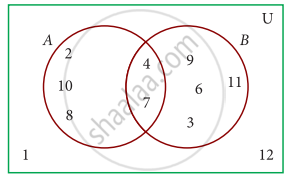Exercise 1.3 | Q 1. (ii) | Page 18

Using the given Venn diagram, write the element of BExercise 1.3 | Q 1. (iii) | Page 18

Using the given Venn diagram, write the element of A ∪ BExercise 1.3 | Q 1. (iv) | Page 18

Using the given Venn diagram, write the element of A ∩ BExercise 1.3 | Q 1. (v) | Page 18

Using the given Venn diagram, write the element of A – BExercise 1.3 | Q 1. (vi) | Page 18

Using the given Venn diagram, write the element of B – AExercise 1.3 | Q 1. (vii) | Page 18

Using the given Venn diagram, write the element of A’Exercise 1.3 | Q 1. (viii) | Page 18

Using the given Venn diagram, write the element of B’Exercise 1.3 | Q 1. (ix) | Page 18

Using the given Venn diagram, write the element of UExercise 1.3 | Q 2. (i) | Page 18

Find A ∪ B, A ∩ B, A – B and B – A for the following sets

A = {2, 6, 10, 14} and B = {2, 5, 14, 16}

Exercise 1.3 | Q 2. (ii) | Page 18

Find A ∪ B, A ∩ B, A – B and B – A for the following sets

A = {a, b, c, e, u} and B = {a, e, i, o, u}

Exercise 1.3 | Q 2. (iii) | Page 18

Find A ∪ B, A ∩ B, A – B and B – A for the following sets

A = {x : x ∈ N, x ≤ 10} and B = {x : x ∈ W, x < 6}

Exercise 1.3 | Q 2. (iv) | Page 18

Find A ∪ B, A ∩ B, A – B and B – A for the following sets

A = Set of all letters in the word “mathematics” and

B = Set of all letters in the word “geometry”

Exercise 1.3 | Q 3. (i) | Page 19

If U = {a, b, c, d, e, f, g, h}, A = {b, d, f, h} and B = {a, d, e, h}, find the following set

A’

Exercise 1.3 | Q 3. (ii) | Page 19

If U = {a, b, c, d, e, f, g, h}, A = {b, d, f, h} and B = {a, d, e, h}, find the following set

B’

Exercise 1.3 | Q 3. (iii) | Page 19

If U = {a, b, c, d, e, f, g, h}, A = {b, d, f, h} and B = {a, d, e, h}, find the following set

A’ ∪ B’

Exercise 1.3 | Q 3. (iv) | Page 19

If U = {a, b, c, d, e, f, g, h}, A = {b, d, f, h} and B = {a, d, e, h}, find the following set

A’ ∩ B’

Exercise 1.3 | Q 3. (v) | Page 19

If U = {a, b, c, d, e, f, g, h}, A = {b, d, f, h} and B = {a, d, e, h}, find the following set

(A ∪ B)’

Exercise 1.3 | Q 3. (vi) | Page 19

If U = {a, b, c, d, e, f, g, h}, A = {b, d, f, h} and B = {a, d, e, h}, find the following set

(A ∩ B)’

Exercise 1.3 | Q 3. (vii) | Page 19

If U = {a, b, c, d, e, f, g, h}, A = {b, d, f, h} and B = {a, d, e, h}, find the following set

(A’)’

Exercise 1.3 | Q 3. (viii) | Page 19

If U = {a, b, c, d, e, f, g, h}, A = {b, d, f, h} and B = {a, d, e, h}, find the following set

(B’)’

Exercise 1.3 | Q 4. (i) | Page 19

Let U = {0, 1, 2, 3, 4, 5, 6, 7}, A = {1, 3, 5, 7} and B = {0, 2, 3, 5, 7}, find the following set

A’

Exercise 1.3 | Q 4. (ii) | Page 19

Let U = {0, 1, 2, 3, 4, 5, 6, 7}, A = {1, 3, 5, 7} and B = {0, 2, 3, 5, 7}, find the following set

B’

Exercise 1.3 | Q 4. (iii) | Page 19

Let U = {0, 1, 2, 3, 4, 5, 6, 7}, A = {1, 3, 5, 7} and B = {0, 2, 3, 5, 7}, find the following set

A‘ ∪ B’

Exercise 1.3 | Q 4. (iv) | Page 19

Let U = {0, 1, 2, 3, 4, 5, 6, 7}, A = {1, 3, 5, 7} and B = {0, 2, 3, 5, 7}, find the following set

A’ ∩ B’

Exercise 1.3 | Q 4. (v) | Page 19

Let U = {0, 1, 2, 3, 4, 5, 6, 7}, A = {1, 3, 5, 7} and B = {0, 2, 3, 5, 7}, find the following set

(A ∪ B)’

Exercise 1.3 | Q 4. (vi) | Page 19

Let U = {0, 1, 2, 3, 4, 5, 6, 7}, A = {1, 3, 5, 7} and B = {0, 2, 3, 5, 7}, find the following set

(A ∩ B)’

Exercise 1.3 | Q 4. (vii) | Page 19

Let U = {0, 1, 2, 3, 4, 5, 6, 7}, A = {1, 3, 5, 7} and B = {0, 2, 3, 5, 7}, find the following set

(A’)’

Exercise 1.3 | Q 4. (viii) | Page 19

Let U = {0, 1, 2, 3, 4, 5, 6, 7}, A = {1, 3, 5, 7} and B = {0, 2, 3, 5, 7}, find the following set

(B’)’

Exercise 1.3 | Q 5. (i) | Page 19

Find the symmetric difference between the following sets

P = {2, 3, 5, 7, 11} and Q = {1, 3, 5, 11}

Exercise 1.3 | Q 5. (ii) | Page 19

Find the symmetric difference between the following sets

R = {l, m, n, o, p} and S = {j, l, n, q}

Exercise 1.3 | Q 5. (iii) | Page 19

Find the symmetric difference between the following sets

X = {5, 6, 7} and Y = {5, 7, 9, 10}

Exercise 1.3 | Q 6. (i) | Page 19

Using the set symbols, write down the expressions for the shaded region in the following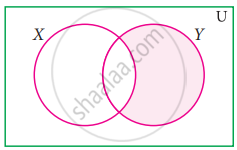Exercise 1.3 | Q 6. (ii) | Page 19

Using the set symbols, write down the expressions for the shaded region in the following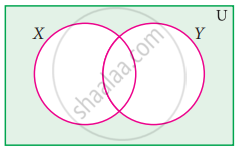Exercise 1.3 | Q 6. (iii) | Page 19

Using the set symbols, write down the expressions for the shaded region in the following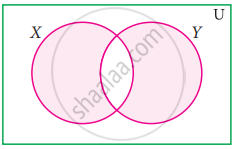Exercise 1.3 | Q 7. (i) | Page 19

Let A and B be two overlapping set and the universal set be U. Draw appropriate Venn diagram for the following

A ∪ B

Exercise 1.3 | Q 7. (ii) | Page 19

Let A and B be two overlapping set and the universal set U. Draw appropriate Venn diagram for the following

A ∩ B

Exercise 1.3 | Q 7. (iii) | Page 19

Let A and B be two overlapping set and the universal set U. Draw appropriate Venn diagram for the following

(A ∩ B)’

Exercise 1.3 | Q 7. (iv) | Page 19

Let A and B be two overlapping set and the universal set U. Draw appropriate Venn diagram for the following

(B – A)’

Exercise 1.3 | Q 7. (v) | Page 19

Let A and B be two overlapping set and the universal set U. Draw appropriate Venn diagram for the following

A’ ∪ B’

Exercise 1.3 | Q 7. (vi) | Page 19

Let A and B be two overlapping set and the universal set U. Draw appropriate Venn diagram for the following

A’ ∩ B’

Exercise 1.3 | Q 7. (vii) | Page 19

Let A and B be two overlapping set and the universal set U. Draw appropriate Venn diagram for the following

What do you observe from (A ∩ B)′ and A′ ∪ B′?

Advertisement Remove all ads
Exercise 1.4 [Page 22]

### Samacheer Kalvi solutions for Mathematics Class 9th Tamil Nadu State Board Chapter 1 Set Language Exercise 1.4 [Page 22]

Exercise 1.4 | Q 1. (i) | Page 22

If P = {1, 2, 5, 7, 9}, Q = {2, 3, 5, 9, 11}, R = {3, 4, 5, 7, 9} and S = {2, 3, 4, 5, 8} then find (P ∪ Q) ∪ R

Exercise 1.4 | Q 1. (ii) | Page 22

If P = {1, 2, 5, 7, 9}, Q = {2, 3, 5, 9, 11}, R = {3, 4, 5, 7, 9} and S = {2, 3, 4, 5, 8} then find (P ∩ Q) ∩ S

Exercise 1.4 | Q 1. (iii) | Page 22

If P = {1, 2, 5, 7, 9}, Q = {2, 3, 5, 9, 11}, R = {3, 4, 5, 7, 9} and S = {2, 3, 4, 5, 8} then find (Q ∩ S) ∩ R

Exercise 1.4 | Q 2 | Page 22

Test for the commutative property of union and intersection of the sets

P = {x : x is a real number between 2 and 7} and

Q = {x : x is an irrational number between 2 and 7}

Exercise 1.4 | Q 3 | Page 22

If A = {p, q, r, s}, B = {m, n, q, s, t} and C = {m, n, p, q, s}, then verify the associative property of union of sets

Exercise 1.4 | Q 4 | Page 22

Verify the associative property of intersection of sets for A = {-11, sqrt(2), sqrt(5), 7}, B = {sqrt(3), sqrt(5), 6, 13} and C = {sqrt(2), sqrt(3), sqrt(5), 9}

Exercise 1.4 | Q 5 | Page 22

If A = {x : x = 2n, n ∈ W and n < 4}, B = {x : x = 2n, n ∈ N and n ≤ 4} and C = {0, 1, 2, 5, 6}, then verify the associative property of intersection of sets

Advertisement Remove all ads
Exercise 1.5 [Page 28]

### Samacheer Kalvi solutions for Mathematics Class 9th Tamil Nadu State Board Chapter 1 Set Language Exercise 1.5 [Page 28]

Exercise 1.5 | Q 1. (i) | Page 28

Using the adjacent Venn diagram, find the following set:

A – B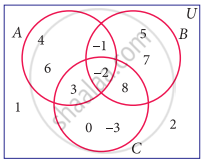Exercise 1.5 | Q 1. (ii) | Page 28

Using the adjacent Venn diagram, find the following set:

B – CExercise 1.5 | Q 1. (iii) | Page 28

Using the adjacent Venn diagram, find the following set:

A’ ∪ B’Exercise 1.5 | Q 1. (iv) | Page 28

Using the adjacent Venn diagram, find the following set:

A’ ∩ B’Exercise 1.5 | Q 1. (v) | Page 28

Using the adjacent Venn diagram, find the following set:

(B ∪ C)’Exercise 1.5 | Q 1. (vi) | Page 28

Using the adjacent Venn diagram, find the following set:

A – (B ∪ C)Exercise 1.5 | Q 1. (vii) | Page 28

Using the adjacent Venn diagram, find the following set:

A – (B ∩ C)Exercise 1.5 | Q 2. (i) | Page 28

If K = {a, b, d, e, f}, L = {b, c, d, g} and M = {a, b, c, d, h} then find the following:

K ∪ (L ∩ M)

Exercise 1.5 | Q 2. (ii) | Page 28

If K = {a, b, d, e, f}, L = {b, c, d, g} and M = {a, b, c, d, h} then find the following:

K ∩ (L ∪ M)

Exercise 1.5 | Q 2. (iii) | Page 28

If K = {a, b, d, e, f}, L = {b, c, d, g} and M = {a, b, c, d, h} then find the following:

(K ∪ L) ∩ (K ∪ M)

Exercise 1.5 | Q 2. (iv) | Page 28

If K = {a, b, d, e, f}, L = {b, c, d, g} and M = {a, b, c, d, h} then find the following:

(K ∩ L) ∪ (K ∩ M) and verify distributive laws

Exercise 1.5 | Q 3 | Page 28

If A = {x : x ∈ Z, −2 < x ≤ 4}, B = {x : x ∈ W, x ≤ 5}, C = {− 4, −1, 0, 2, 3, 4} verify A ∪ (B ∩ C) = (A ∪ B) ∩ (A ∪ C)

Exercise 1.5 | Q 4 | Page 28

Verify A ∪ (B ∩ C) = (A ∪ B) ∩ (A ∪ C) using Venn diagrams

Exercise 1.5 | Q 5 | Page 28

If A = {b, c, e, g, h}, B = {a, c, d, g, f}, and C = {a, d, e, g, h}, then show that A – (B ∩ C) = (A – B) ∪ (A – C)

Exercise 1.5 | Q 6 | Page 28

If A = {x : x = 6n, n ∈ W and n < 6}, B = {x : x = 2n, n ∈ N and 2 < n ≤ 9} and C = {x : x = 3n, n ∈ N and 4 ≤ n < 10}, then show that A – (B ∩ C) = (A – B) ∪ (A – C)

Exercise 1.5 | Q 7 | Page 28

If A = {– 2, 0, 1, 3, 5}, B = {–1, 0, 2, 5, 6} and C = {–1, 2, 5, 6, 7}, then show that A – (B ∪ C) = (A – B) ∩ (A – C)

Exercise 1.5 | Q 8 | Page 28

If A = {y : y = ("a"+1)/2, "a" ∈ "W" and "a" ≤ 5}, B = {y : y = (2"n" – 1)/2, "n" ∈ "W" and "n" < 5} and C = {-1, −1/2, 1, 3/2, 2} then show that A – (B ∪ C) = (A – B) ∩ (A – C)

Exercise 1.5 | Q 9 | Page 28

Verify A – (B ∩ C) = (A – B) ∪ (A – C) using Venn diagrams

Exercise 1.5 | Q 10 | Page 28

If U = {4, 7, 8, 10, 11, 12, 15, 16}, A = {7, 8, 11, 12} and B = {4, 8, 12, 15}, then verify De Morgan’s Laws for complementation

Exercise 1.5 | Q 11 | Page 28

Verify (A ∩ B)’ = A’ ∪ B’ using Venn diagrams

Advertisement Remove all ads
Exercise 1.6 [Pages 35 - 36]

### Samacheer Kalvi solutions for Mathematics Class 9th Tamil Nadu State Board Chapter 1 Set Language Exercise 1.6 [Pages 35 - 36]

Exercise 1.6 | Q 1. (i) | Page 35

If n(A) = 25, n(B) = 40, n(A ∪ B) = 50 and n(B’) = 25, find n(A ∩ B) and n(U).

Exercise 1.6 | Q 1. (ii) | Page 35

If n(A) = 300, n(A ∪ B) = 500, n(A ∩ B) = 50 and n(B’) = 350, find n(B) and n(U)

Exercise 1.6 | Q 2 | Page 35

If U = {x : x ∈ N, x ≤ 10}, A = {2, 3, 4, 8, 10} and B = {1, 2, 5, 8, 10}, then verify that n(A ∪ B) = n(A) + n(B) – n(A ∩ B)

Exercise 1.6 | Q 3. (i) | Page 35

Verify n(A ∪ B ∪ C) = n(A) + n(B) + n(C) – n(A ∩ B) – n(B ∩ C) – n(A ∩ C) + n(A ∩ B ∩ C) for the following sets

A = {a, c, e, f, h}, B = {c, d, e, f} and C = {a, b, c, f}

Exercise 1.6 | Q 3. (ii) | Page 35

Verify n(A ∪ B ∪ C) = n(A) + n(B) + n(C) – n(A ∩ B) – n(B ∩ C) – n(A ∩ C) + n(A ∩ B ∩ C) for the following sets

A = {1, 3, 5}, B = {2, 3, 5, 6}, C = {1, 5, 6, 7}

Exercise 1.6 | Q 4. (i) | Page 35

In a class, all students take part in either music or drama or both. 25 students take part in music, 30 students take part in drama and 8 students take part in both music and drama. Find the number of students who take part in only music

Exercise 1.6 | Q 4. (ii) | Page 35

In a class, all students take part in either music or drama or both. 25 students take part in music, 30 students take part in drama and 8 students take part in both music and drama. Find the number of students who take part in only drama

Exercise 1.6 | Q 4. (iii) | Page 35

In a class, all students take part in either music or drama or both. 25 students take part in music, 30 students take part in drama and 8 students take part in both music and drama. Find the total number of students in the class

Exercise 1.6 | Q 5. (i) | Page 35

In a party of 45 people, each one likes tea or coffee or both. 35 people like tea and 20 people like coffee. Find the number of people who like both tea and coffee

Exercise 1.6 | Q 5. (ii) | Page 35

In a party of 45 people, each one likes tea or coffee or both. 35 people like tea and 20 people like coffee. Find the number of people who do not like tea

Exercise 1.6 | Q 5. (iii) | Page 35

In a party of 45 people, each one likes tea or coffee or both. 35 people like tea and 20 people like coffee. Find the number of people who do not like coffee

Exercise 1.6 | Q 6 | Page 35

In an examination 50% of the students passed in Mathematics and 70% of students passed in Science while 10% students failed in both subjects. 300 students passed in both the subjects. Find the total number of students who appeared in the examination, if they took examination in only two subjects

Exercise 1.6 | Q 7 | Page 35

A and B are two sets such that n(A – B) = 32 + x, n(B – A) = 5x and n(A ∩ B) = x. Illustrate the information by means of a Venn diagram. Given that n(A) = n(B). Calculate the value of x

Exercise 1.6 | Q 8 | Page 35

Out of 500 car owners investigated, 400 owned car A and 200 owned car B, 50 owned both A and B cars. Is this data correct?

Exercise 1.6 | Q 9. (i) | Page 35

In a colony, 275 families buy Tamil newspaper, 150 families buy English newspaper, 45 families buy Hindi newspaper, 125 families buy Tamil and English newspaper, 17 families buy English and Hindi newspapers, 5 families buy Tamil and Hindi newspaper and 3 families buy all the three newspaper. If each family buy atleast one of these newspaper then find Number of families buy only one newspaper

Exercise 1.6 | Q 9. (ii) | Page 35

In a colony, 275 families buy Tamil newspaper, 150 families buy English newspaper, 45 families buy Hindi newspaper, 125 families buy Tamil and English newspaper, 17 families buy English and Hindi newspapers, 5 families buy Tamil and Hindi newspaper and 3 families buy all the three newspaper. If each family buy atleast one of these newspaper then find Number of families buy atleast two newspaper

Exercise 1.6 | Q 9. (iii) | Page 35

In a colony, 275 families buy Tamil newspaper, 150 families buy English newspaper, 45 families buy Hindi newspaper, 125 families buy Tamil and English newspaper, 17 families buy English and Hindi newspapers, 5 families buy Tamil and Hindi newspaper and 3 families buy all the three newspaper. If each family buy atleast one of these newspaper then find Total number of families in the colony

Exercise 1.6 | Q 10 | Page 36

A survey of 1000 farmers found that 600 grew paddy, 350 grew ragi, 280 grew corn, 120 grew paddy and ragi, 100 grew ragi and corn, 80 grew paddy and corn. If each farmer grew atleast anyone of the above three, then find the number of farmers who grew all the three.

Exercise 1.6 | Q 11 | Page 36

In the adjacent diagram, if n(U) = 125, y is two times of x and z is 10 more than x, then find the value of x, y and z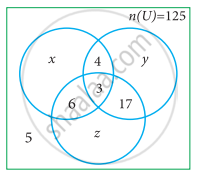Exercise 1.6 | Q 12. (i) | Page 36

Each student in a class of 35 plays atleast one game among chess, carrom and table tennis. 22 play chess, 21 play carrom, 15 play table tennis, 10 play chess and table tennis, 8 play carrom and table tennis and 6 play all the three games. Find the number of students who play chess and carrom but not table tennis (Hint: Use Venn diagram)

Exercise 1.6 | Q 12. (ii) | Page 36

Each student in a class of 35 plays atleast one game among chess, carrom and table tennis. 22 play chess, 21 play carrom, 15 play table tennis, 10 play chess and table tennis, 8 play carrom and table tennis and 6 play all the three games. Find the number of students who play only chess (Hint: Use Venn diagram)

Exercise 1.6 | Q 12. (iii) | Page 36

Each student in a class of 35 plays atleast one game among chess, carrom and table tennis. 22 play chess, 21 play carrom, 15 play table tennis, 10 play chess and table tennis, 8 play carrom and table tennis and 6 play all the three games. Find the number of students who play only carrom (Hint: Use Venn diagram)

Exercise 1.6 | Q 13 | Page 36

In a class of 50 students, each one come to school by bus or by bicycle or on foot. 25 by bus, 20 by bicycle, 30 on foot and 10 students by all the three. Now how many students come to school exactly by two modes of transport?

Advertisement Remove all ads
Exercise 1.7 [Pages 36 - 37]

### Samacheer Kalvi solutions for Mathematics Class 9th Tamil Nadu State Board Chapter 1 Set Language Exercise 1.7 [Pages 36 - 37]

#### Multiple Choice Questions

Exercise 1.7 | Q 1 | Page 36

Which of the following is correct?

• {7} ∈ {1, 2, 3, 4, 5, 6, 7, 8, 9, 10}

• 7 ∈ {1, 2, 3, 4, 5, 6, 7, 8, 9, 10}

• 7 ∉ {1, 2, 3, 4, 5, 6, 7, 8, 9, 10}

• {7} ⊄ {1, 2, 3, 4, 5, 6, 7, 8, 9, 10}

Exercise 1.7 | Q 2 | Page 36

The set P = {x | x ∈ Z, −1 < x <1} is a

• Singleton set

• Power set

• Null set

• Subset

Exercise 1.7 | Q 3 | Page 36

If U = {x | x ∈ N, x < 10} and A = {x | x ∈ N, 2 ≤ x < 6} then (A’)’ is

• {1, 6, 7, 8, 9}

• {1, 2, 3, 4}

• {2, 3, 4, 5}

• { }

Exercise 1.7 | Q 4 | Page 36

If B ⊆ A then n(A ∩ B) is

• n(A – B)

• n(B)

• n(B – A)

• n(A)

Exercise 1.7 | Q 5 | Page 36

If A = {x, y, z} then the number of non-empty subsets of A is

• 8

• 5

• 6

• 7

Exercise 1.7 | Q 6 | Page 37

Which of the following is correct?

• Ø ⊆ {a, b}

• Ø ∈ {a, b}

• {a} ∈ {a, b}

• a ⊆ {a, b}

Exercise 1.7 | Q 7 | Page 37

If A ∪ B = A ∩ B then

• A ≠ B

• A = B

• A ⊂ B

• B ⊂ A

Exercise 1.7 | Q 8 | Page 37

If B – A is B, then A ∩ B is

• A

• B

• U

• Ø

Exercise 1.7 | Q 9 | Page 37

From the adjacent diagram n[P(AΔB)] is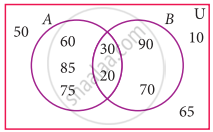• 8

• 16

• 32

• 64

Exercise 1.7 | Q 10 | Page 37

If n(A) = 10 and n(B) = 15, then the minimum and maximum number of elements in A ∩ B is

• 10, 15

• 15, 10

• 10, 0

• 0, 10

Exercise 1.7 | Q 11 | Page 37

Let A = {Ø} and B = P(A) then A ∩ B is

• {Ø, {Ø}}

• {Ø}

• Ø

• {0}

Exercise 1.7 | Q 12 | Page 37

In a class of 50 boys, 35 boys play Carrom and 20 boys play Chess then the number of boys play both games is

• 5

• 30

• 15

• 10

Exercise 1.7 | Q 13 | Page 37

If U = {x : x ∈ N and x < 10}, A = {1, 2, 3, 5, 8} and B = {2, 5, 6, 7, 9}, then n[(A ∪ B)’] is

• 1

• 2

• 4

• 8

Exercise 1.7 | Q 14 | Page 37

For any three sets P, Q and R, P – (Q ∩ R) is

• P – (Q ∪ R)

• (P ∩ Q) – R

• (P – Q) ∪ (P – R)

• (P – Q) ∩ (P – R)

Exercise 1.7 | Q 15 | Page 37

Which of the following is true?

• A – B = A ∩ B

• A – B = B – A

• (A ∪ B)’ = A’ ∪ B’

• (A ∩ B)’ = A’ ∪ B’

Exercise 1.7 | Q 16 | Page 37

If n(A ∪ B ∪ C) = 100, n(A) = 4x, n(B) = 6x, n(C) = 5x, n(A ∩ B) = 20, n(B ∩ C) = 15, n(A ∩ C) = 25 and n(A ∩ B ∩ C) = 10, then the value of x is

• 10

• 15

• 25

• 30

Exercise 1.7 | Q 17 | Page 37

For any three sets A, B and C, (A – B) ∩ (B – C) is equal to

• A only

• B only

• C only

• ϕ

Exercise 1.7 | Q 18 | Page 37

If J = Set of three sided shapes, K = Set of shapes with two equal sides and L = Set of shapes with right angle, then J ∩ K ∩ L is

• Set of isosceles triangles

• Set of equilateral triangles

• Set of isosceles right triangles

• Set of right angled triangles

Exercise 1.7 | Q 19 | Page 37

The shaded region in the Venn diagram is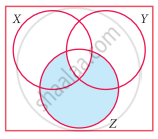• Z – (X ∪ Y)

• (X ∪ Y) ∩ Z

• Z – (X ∩ Y)

• Z ∪ (X ∩ Y)

Exercise 1.7 | Q 20 | Page 37

In a city, 40% people like only one fruit, 35% people like only two fruits, 20% people like all the three fruits. How many percentage of people do not like any one of the above three fruits?

• 5

• 8

• 10

• 15

Advertisement Remove all ads

## Chapter 1: Set Language

Exercise 1.1Exercise 1.2Exercise 1.3Exercise 1.4Exercise 1.5Exercise 1.6Exercise 1.7## Samacheer Kalvi solutions for Mathematics Class 9th Tamil Nadu State Board chapter 1 - Set Language

Samacheer Kalvi solutions for Mathematics Class 9th Tamil Nadu State Board chapter 1 (Set Language) include all questions with solution and detail explanation. This will clear students doubts about any question and improve application skills while preparing for board exams. The detailed, step-by-step solutions will help you understand the concepts better and clear your confusions, if any. Shaalaa.com has the Tamil Nadu Board of Secondary Education Mathematics Class 9th Tamil Nadu State Board solutions in a manner that help students grasp basic concepts better and faster.

Further, we at Shaalaa.com provide such solutions so that students can prepare for written exams. Samacheer Kalvi textbook solutions can be a core help for self-study and acts as a perfect self-help guidance for students.

Concepts covered in Mathematics Class 9th Tamil Nadu State Board chapter 1 Set Language are Concept of Sets, Representation of a Set, Types of Sets, Set Operations, Properties of Set Operations, De Morgan’s Laws, Cardinality of a Set.

Using Samacheer Kalvi Class 9th solutions Set Language exercise by students are an easy way to prepare for the exams, as they involve solutions arranged chapter-wise also page wise. The questions involved in Samacheer Kalvi Solutions are important questions that can be asked in the final exam. Maximum students of Tamil Nadu Board of Secondary Education Class 9th prefer Samacheer Kalvi Textbook Solutions to score more in exam.

Get the free view of chapter 1 Set Language Class 9th extra questions for Mathematics Class 9th Tamil Nadu State Board and can use Shaalaa.com to keep it handy for your exam preparation

Advertisement Remove all ads
Share
Notifications

View all notifications

Forgot password?